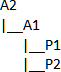# Equations in Assemblies

The equation syntax for references between assembly components loads automatically when you select dimensions, features, and global variables in the FeatureManager design tree, the graphics area, File Properties, and the Equations dialog box.

Existing equations that do not follow this syntax are marked as errors because they create inconsistent results. You need to edit these equations to correct the syntax.

In the following example, A1 is a subassembly of A2 containing the parts P1 and P2:GV1 and GV2 are global variables in the documents. Use the following syntax:

Edit Target Reference to Syntax
A2 A2 “GV1” = “GV2”
A2 A1 “GV1” = “GV2@A1<1>.Assembly
A2 P1 “GV1” = “GV2@P1<1>.Part@A1<1>.Assembly
A1 A2 “GV1” = “GV2@A2.Assembly”
A1 A1 “GV1” = “GV2”
A1 P1 “GV1” = “GV2@P1<1>.Part”
P1 A2 “GV1” = “GV2@A2.Assembly”
P1 A1 “GV1” = “GV2@A1<1>.Assembly”
P1 P1 “GV1” = “GV2”
P1 P2 “GV1” = “GV2@P2<1>.Part@A1<1>.Assembly”